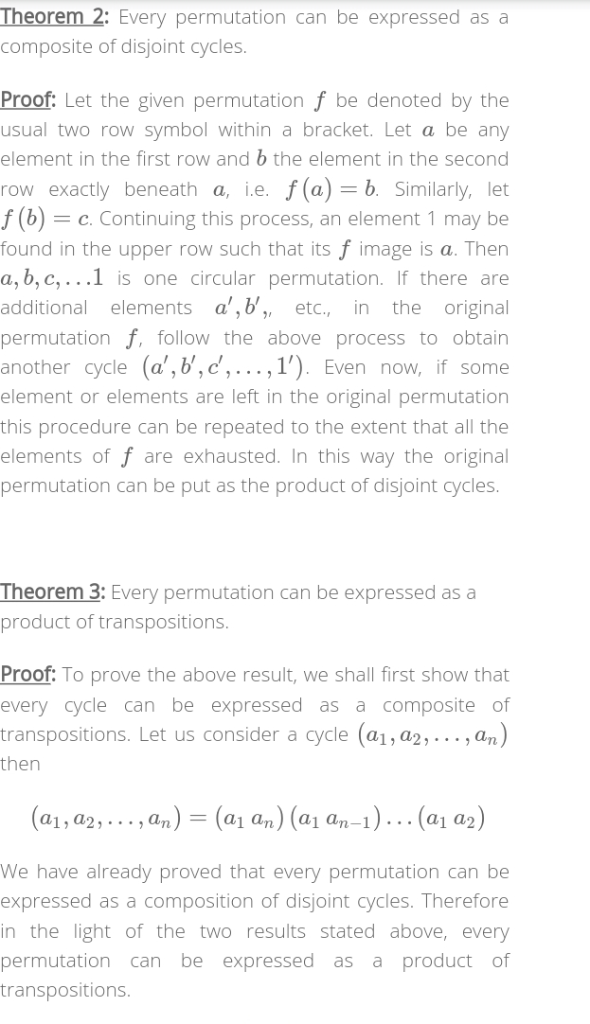Homework Help Question & Answers

Explain why every permutation in S(n) can be represented by a product of n-1 or fewer cycles of length 2 (transpositions). Represent the permutationσ in problem (1) above as a product of 8 or fewer tr...

Explain why every permutation in S(n) can be represented by a product of n-1 or fewer cycles of length 2 (transpositions). Represent the permutationσ in problem (1) above as a product of 8 or fewer transpositions. Is σ an even or an odd permuation?Add Answer of: Explain why every permutation in S(n) can be represented by a product of n-1 or fewer cycles of length 2 (transpositions). Represent the permutationσ in problem (1) above as a product of 8 or fewer tr...
More Homework Help Questions Additional questions in this topic.

• 3. Assuming atoms can be represented as hard spheres, the bonding energy between a sodium ion and a chloride ion pair can be represented by: 1.436 7.32x10-6 8 1 1 where U is energy per ion pair in eV...

Need Online Homework Help?

Get FREE EXPERT Answers
WITHIN MINUTES
Related Questions